Elementary Math Ratio and proportion

# Ratio and proportion

Here you will find all the elementary ratio and proportion resources you need. Like all our elementary math topic guides, we’ve included step-by-step guides, detailed examples and practice questions!

Filter by:

Converting fractions decimals and percents

Percent

Show all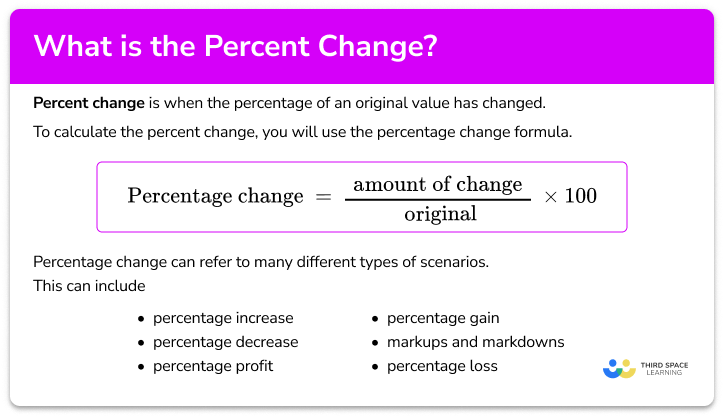Percent change

Percent change step-by-step topic guide, detailed examples, practice questions, teaching tips and FAQs.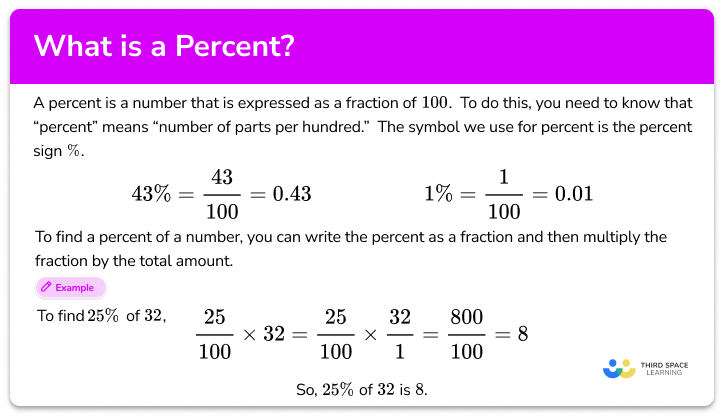Percent

Percent step-by-step topic guide, detailed examples, practice questions, teaching tips and FAQs.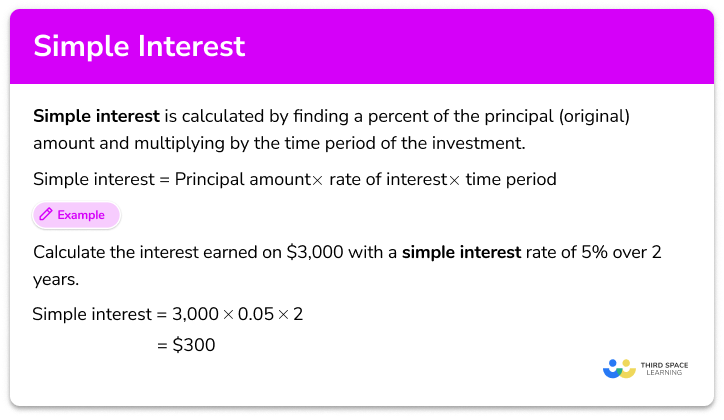Simple interest

Simple interest step-by-step topic guide, detailed examples, practice questions, teaching tips and FAQs.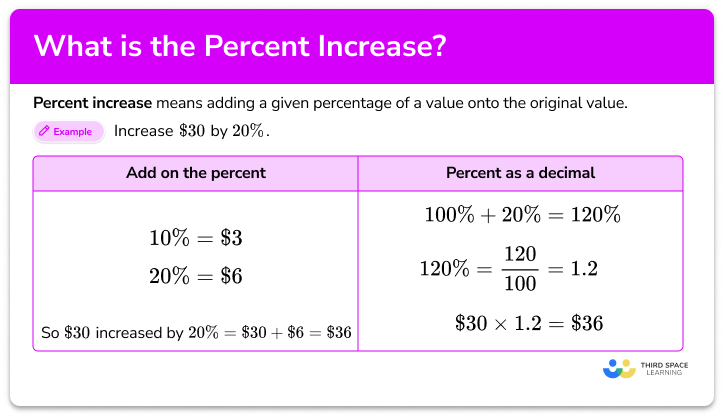Percent increase

Percent increase step-by-step topic guide, detailed examples, practice questions, teaching tips and FAQs.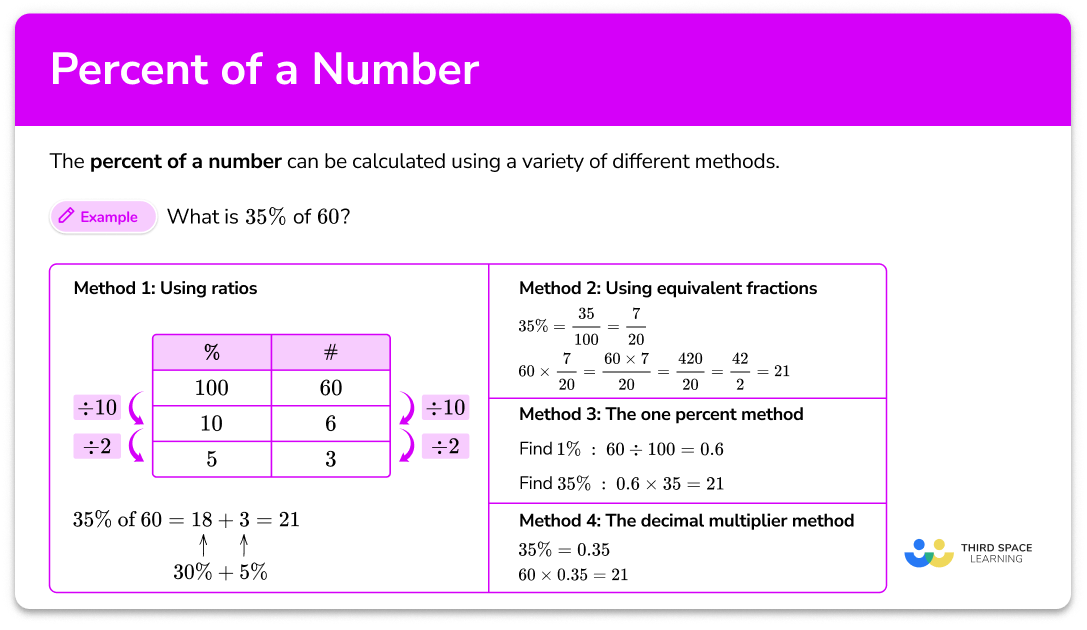Percent of a number

Percent of a number step-by-step topic guide, detailed examples, practice questions, teaching tips and FAQs.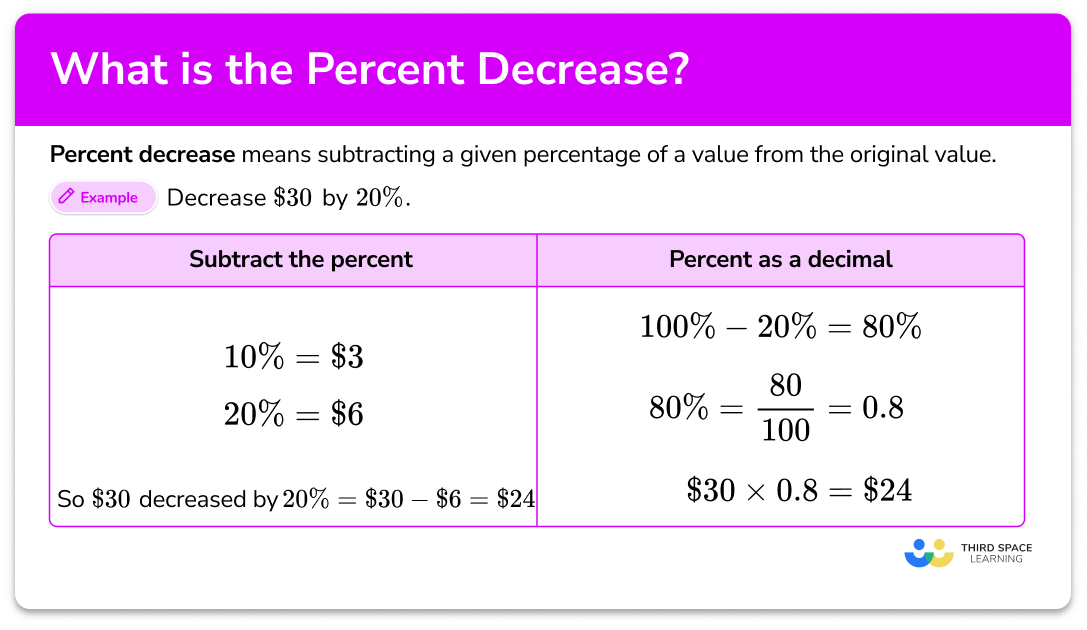Percent decrease

Percent decrease step-by-step topic guide, detailed examples, practice questions, teaching tips and FAQs.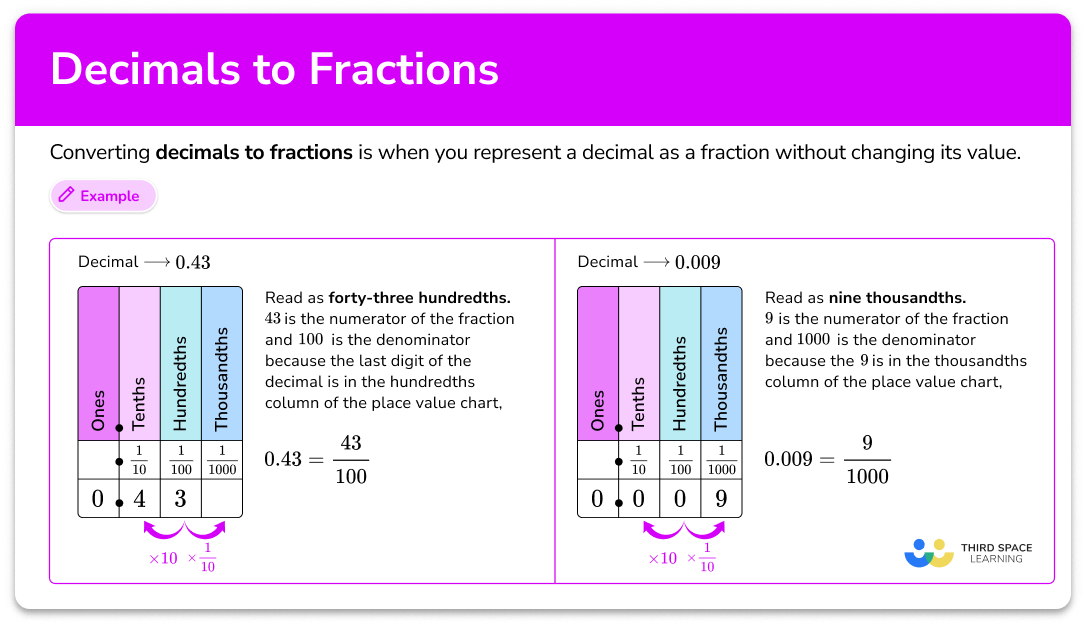Decimal to fraction

Decimals to fractions step-by-step topic guide, detailed examples, practice questions, teaching tips and FAQs.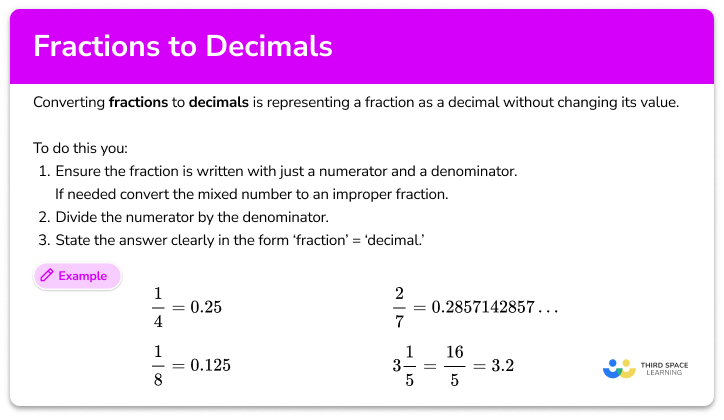Fraction to decimal

Fractions to decimals step-by-step topic guide, detailed examples, practice questions, teaching tips and FAQs.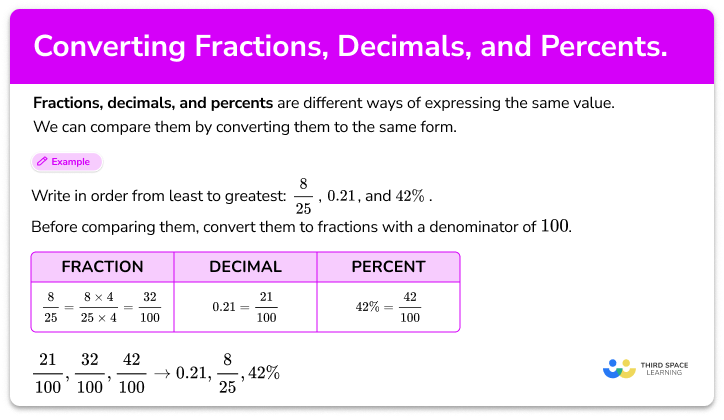Converting fractions, decimals and percentages

Converting fractions, decimals and percentages step-by-step topic guide, detailed examples, practice questions, teaching tips and FAQs.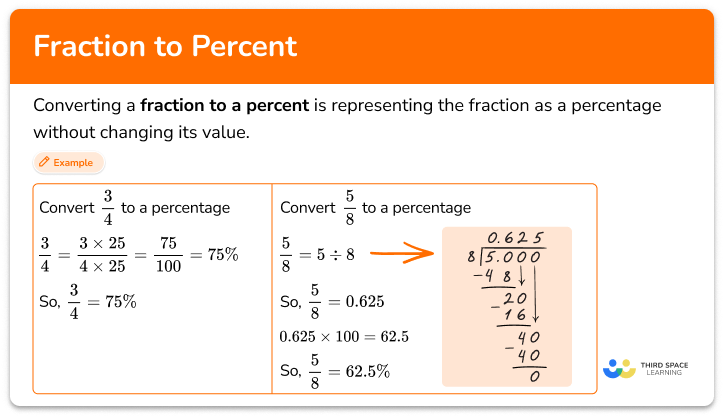Fraction to percent

Fraction to percent step-by-step topic guide, detailed examples, practice questions, teaching tips and FAQs.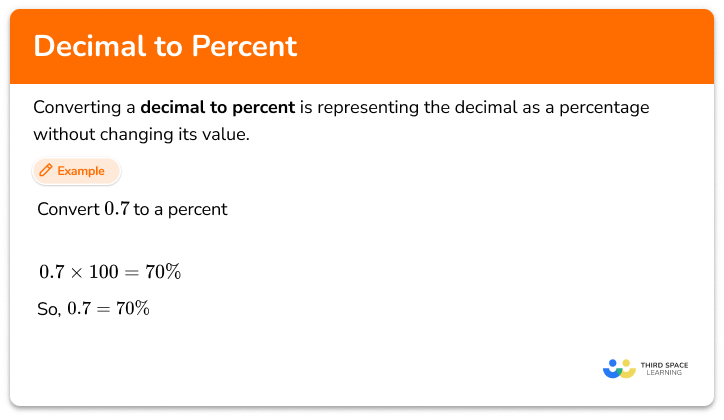Decimal to percent

Decimal to percent step-by-step topic guide, detailed examples, practice questions, teaching tips and FAQs.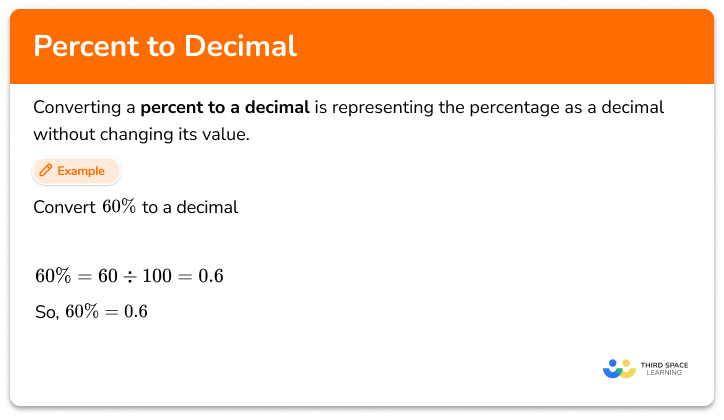Percent to decimal

Percent to decimal step-by-step topic guide, detailed examples, practice questions, teaching tips and FAQs.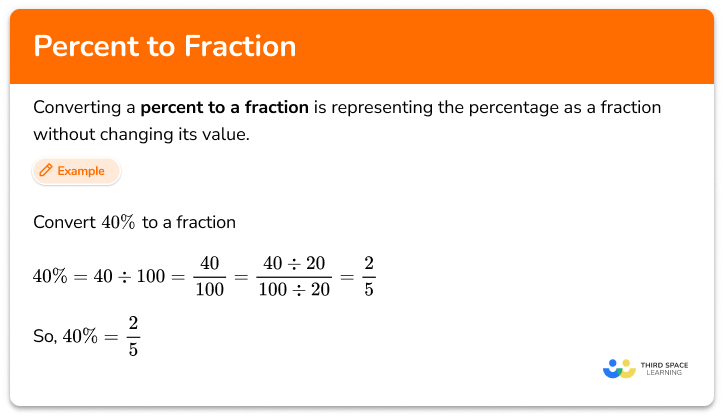Percent to fraction

Percent to fraction step-by-step topic guide, detailed examples, practice questions, teaching tips and FAQs.